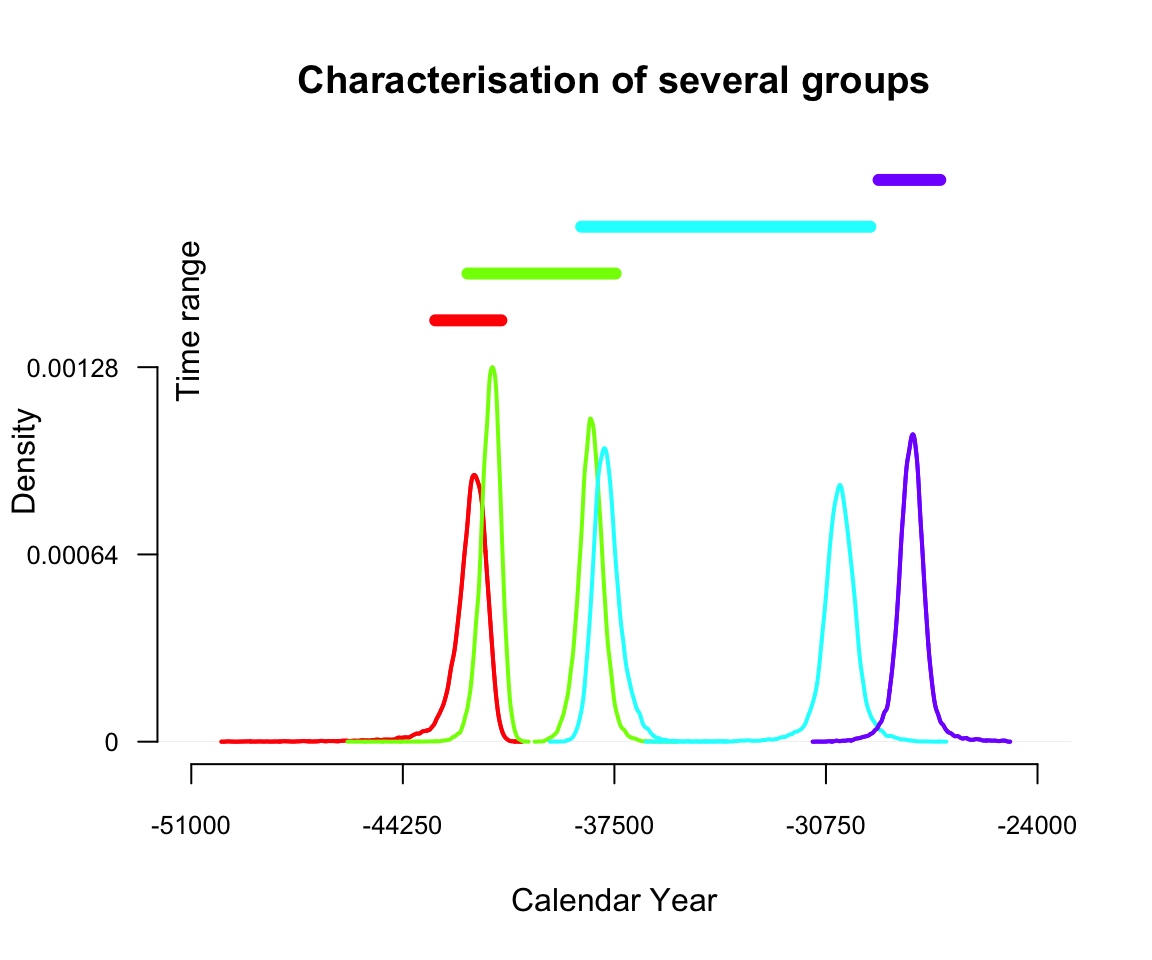# 3. ArchaeoPhases : Analysis of groups of dates

## Groups of dates

A group of dates is defined by the date of the minimum and the date of the maximum of the group. In this part, we will use the data containing these values for each group of dates.

### Time Range Interval

We can estimate the time range of a group of dates as the shortest interval that contains all the dates of the group at a given confidence level (see Philippe and Vibet 2017 for more details). The following code gives the endpoints of the time range of all groups of dates of Ksar Akil data and recall the given confidence level.

``````data("KAPhasesChronoModel")
MultiPhaseTimeRange(KAPhasesChronoModel, c(8,6,4,2), level = 0.95)``````
``````                             Level TimeRangeInf TimeRangeSup
IUP.alpha IUP.beta            0.95       -43217       -41106
[ getOption("max.print") est atteint -- 3 lignes omises ]``````

The time range interval of the group of dates is a way to summarise the estimation of its minimum, the estimation of its maximum and their uncertainties at the same time.

### Graphical representation

The function PhasePlot() may be used to draw a plot of the characteristics of several groups of dates on a same graph : the marginal posterior density of the minimum and the maximum of each group and its time range at a desired level.

``````data("KAPhasesChronoModel")
MultiPhasePlot(KAPhasesChronoModel, c(8,6,4,2), level = 0.95)``````## Succession of groups

We may also be interested in a succession of phases. This is actually the case of the succession of IUP, Ahmarian, UP and EPI that are in stratigraphic order. Hence, we can estimate the transition interval and, if it exists, the gap between these successive phases.

### Transistions between successive groups

The transition interval between two successive phases is the shortest interval that covers the end of the oldest group of dates and the start of the youngest group of dates. The start and the end are estimated by the minimum and the maximum of the dates included in the group of dates. It gives an idea of the transition period between two successive group of dates. From a computational point of view this is equivalent to the time range calculated between the end of the oldest group of dates and the start of the youngest group of dates. See Philippe and Vibet 2017 for more statistical details.

``````data("KAPhasesChronoModel")
MultiPhasesTransition(KAPhasesChronoModel, c(8,6,4,2), level = 0.95)``````
``````                          0.95 TransitionRangeInf TransitionRangeSup
IUP.beta & Ahmarian.alpha 0.95             -43241             -40728
[ getOption("max.print") est atteint -- 2 lignes omises ]``````

For this function, the order of the groups of dates is important. The vector of positions of the minimums should start with the minimum of the oldest phase and end with the one of the youngest phase. For data extracted from ChronoModel or using the function CreateMinMaxGroup(), the vector of positions of the phases’ maximums is deduced from the vector of the minimum. For other data, this vector should be specified.

### Gap between successive groups

Successive phases may also be separated in time. Indeed there may exist a gap between them. This testing procedure check whether a gap exists between two successive groups of dates with fixed probability. If a gap exists, it is an interval that covers the end of one group of dates and the start of the successive one with fixed posterior probability. See Philippe and Vibet 2017 for more statistical details.

``````data("KAPhasesChronoModel")
MultiPhasesGap(KAPhasesChronoModel, c(8,6,4,2), level = 0.95)``````
``````                          Level  HiatusIntervalInf HiatusIntervalSup
IUP.beta & Ahmarian.alpha "0.95" "NA"              "NA"
[ getOption("max.print") est atteint -- 2 lignes omises ]``````

At a confidence level of 95%, there is no gap between the succession of phases IUP, Ahmarian and UP, but there exists one of 203 years between phase UP and phase EPI.

### Graphical representation

Now, let’s summarise these pieces of information in a plot. The following lines generate the plot of the succession of phases from Ksar Akil.

``````data("KAPhasesChronoModel")
MultiSuccessionPlot(KAPhasesChronoModel, c(8,6,4,2), level = 0.95)``````